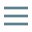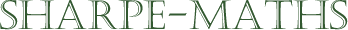Previous topic:
Substitution
Current topic:
Formulas (Transposition)
Next topic:
Glossary of mathematical terms

### Transposition or changing the subject of a formula

This topic can be seen as an extension of the work we have done on solving linear equations and gives us the tools we need to work with formulas of all kinds.

In this section, we will look at various techniques for taking a formula and manipulating it so that we can use it to find other variables.

For example, here is a very simple and very profound formula: $F=ma$. This formula is one of the mainstays of physics and is the core principle behind Newton's second law of motion. This formula allows us to find the Force acting on a body when the mass of the body is $m$ and its acceleration is $a$.

Transposition will allow us to create formulas, where given the values of $m$ and $F$, we can find $a$ or if we are given the values of $F$ and $a$, we can find the value of $m$.